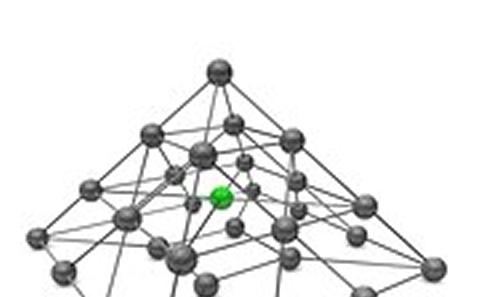The University of Southampton
Mathematical Sciences

# Research project: Decision Making Under Uncertainty

Currently Active:
Yes

Our work includes developing and/or investigating new mathematical models which capture the uncertainty and other features such as competition, equilibrium and hierarchical relationships between decision makers. Typical examples are stochastic principal agent models (Stackelberg leader follower models), stochastic Nash equilibrium models, stochastic bilevel programming models, stochastic mathematical programs with equilibrium constraints (SMPEC) models and stochastic equilibrium programs with equilibrium constraints (SEPEC) models.## Distributionally Robust Optimisation With Matrix Moment Constraints

One of the most challenging issues in decision analysis is to find an optimal decision under uncertainty. The situation can become far more complex if the decision maker does not have complete information on the distribution of the random variables. The project focuses on a class of distributionally robust optimization (DRO) problems where the set of distributions is estimated through moment of random matrices which capture some partial information such as the mean value, the standard deviation or the correlation of the random variables. By converting the DRO problems into mathematical programs with semi-infinite and semi-definite constraints (MPSISDC), the project investigates effective numerical schemes and underlying theory for solving (MPSISDC). This project is funded by the EPSRC.## Global Optimisation of Noisy Functions

We consider global optimisation problems arising from the calibration of financial models to market data, where the underlying model prices are approximated by Monte Carlo simulations. Financial derivatives play an essential role in today’s financial industry and are typically   valuated by means of sophisticated mathematical pricing models. The calibration of these models to market prices, i.e. the estimation of appropriate model parameters, is a crucial procedure for making them applicable to real markets which requires advanced optimisation   techniques. Our approach considers extending classical radial basis function methods for deterministic objective functions to account for   a noisy setup in which the level of noise can be controlled adequately.   Our work is supported by DEVnet GmbH, Germany, a leading service provider in Business Analytics and Data Value Chains.

### Related research groups

Operational Research
Computational Optimisation